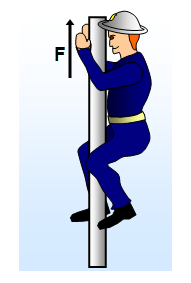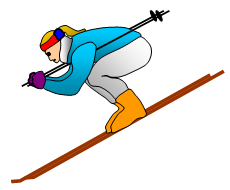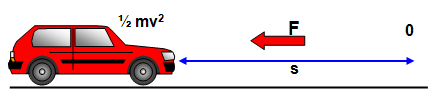# Force, work energy and power – equations

Work done = Energy transformed = Force x displacement = FscosA

(This is an example of two vectors being multiplied together to give a scalar)

### Fire-fighter sliding down a polemgh – ˝mv2 = Fh

mgh – ˝mv2 = Fh

### Parachutist

mgh – ˝mv2 = Fh

with the parachute open the parachutist falls at their terminal velocity and so:

At terminal velocity:      Drag force (F) = mg

### Skier on a slopemgh – ˝mv2 = Fs = Fsin A

where A is the angle of the slope with the horizontal

### Car braking to a stop

˝mv2 = FsPower = Work done/Time taken = Fs/t = Fv

### Useful summary of similar equations involving Force

Impulse = Ft = Force x time
Work = Fs = Force x displacement
Power = Fv = Force x velocity

A VERSION IN WORD IS AVAILABLE ON THE SCHOOLPHYSICS USB